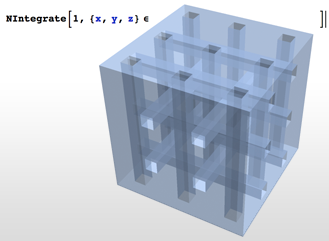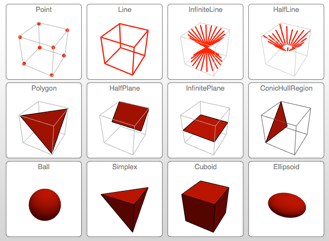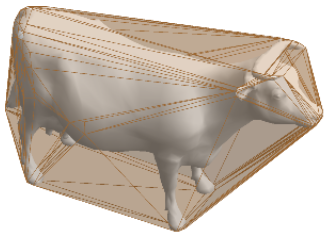# 幾何学的計算

## 記号幾何学 »

Version 10 adds broad support for geometric computation, with the key element being that of geometric region. Geometric regions can be created by using special regions such as circle, using formulas, using meshes containing collections of simple regions, or combining other regions through Boolean combinations and transformations. Several standard properties can be directly computed for every region, such as membership tests, measure (e.g. length, area, volume), centroid, nearest points, etc. Regions can also be used as specifications for many high-level solvers, including optimization, algebraic equation solving, integration, and partial differential equation solving.## 名前付き領域と数式定義領域 »

Version 10 adds full support for basic and formula-based geometric regions. Basic regions are common and easy to specify. Formula regions are flexible and have great descriptive power. Basic and formula regions can be used to compute exact or approximate solutions or results involving parameters, often in any number of dimensions. Basic and formula regions fully support the geometric region framework, including computing properties (area, nearest point, etc.), being used as input to solvers (optimization, solving PDEs, etc.), and discretization.## メッシュベースの領域 »

Version 10 adds full support for mesh-based geometric regions. Mesh-based regions can be explicitly specified or automatically generated from lists of points, from graphics, or from other regions. Mesh-based regions are flexible enough to approximate any other region and support fast algorithms for essentially any operation. The mesh-based regions fully support the geometric region framework, including computing properties (area, nearest point, etc.) and being used as inputs to solvers (integration, solving PDEs, etc.).## Mathematica

Questions? Comments? Contact a Wolfram expert »